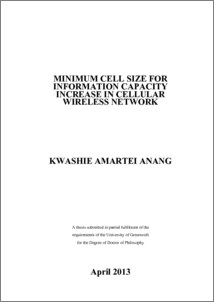# Minimum cell size for information capacity increase in cellular wireless network

Anang, Kwashie Amartei (2013) Minimum cell size for information capacity increase in cellular wireless network. PhD thesis, University of Greenwich.Preview
PDF
Kwashie_Amartei_Anang_2013.pdf - Published Version

## Abstract

In conventional cellular wireless communication system, interference modelling has focused on the six primary co-channel interfering cells (first tier co-channel cells). In the current accepted interference model, co-channel interfering cells beyond the first tier (subsequent tier co-channel cells) are neglected. This currently accepted interference models is suitable for cellular wireless communication systems operating at carrier frequencies, f c = 0.9 and 1.8 GHz, cell size radii R > 1 km and basic path loss exponent α ≥ 2. The future and emerging wireless communication systems are expected to be operating at frequencies f c > 2 GHz (3.35 - 15.75 GHz), cell size radii R≤ 1 km and basic path loss exponent α ≤ 2. This, makes the current acceptable co-channel interference model unsuitable for information capacity analysis of the future cellular systems. Therefore, a co-channel interference model suitable for future and emerging wireless communication system becomes necessary.

In this thesis a new and modified interference model is proposed. The proposed interference model includes the first and subsequent tier co-channel interfering cells. The proposed interference model will be suitable for cellular wireless communication systems operating at carrier frequencies f c > 2 GHz, cell size radii R≤ 1 km and basic path loss exponent α ≤ 2. A mathematical analysis, supported by computer simulation is used, to study the uplink information capacity performance for the conventional and proposed interference model. The analysis and simulation results of the proposed interference model show that at carrier frequencies f c > 2 GHz, co-channel interfering cells beyond the first tier become active as cell size radius R, reduces. As an example for a carrier frequency f c = 15.75 GHz, cell size radius R = 100 m at a normalized reuse distance Ru = 4, there was a 15.32 % decrease in the information capacity between the conventional and proposed interference model.

An information capacity - cost analysis is used to find a minimum cell size for information capacity increase in cellular wireless network, thus a theoretical limit to cell size reduction. The results show that as the cell size radius R reduces to 300 m and less, the proposed interference model show a 5.76 - 18.89 % decrease in the information capacity per unit cost (£, \$, etc) at microwave carrier frequencies f c > 3.35 GHz. This result illustrates that there is a theoretical limit to cell size reduction in relation to information capacity performance and cost.

An inductive approach is used to generate a formula for calculating the number of co-channel interfering cells Nn in a cellular wireless site layout. Such a formula allows one to calculate the number of co-channel interfering cells in subsequent tiers of a cellular wireless site layout. The geometric derivation shows that the number of co-channel interfering cell Nn in a subsequent tier is the product of the number of co-channel interfering cells in the first tier NI and the tier number n. Thus, the number of co-channel interfering cell in a subsequent tier Nn = NI × n. This formula enables subsequent tier co-channel interference to be included in the information capacity analysis of future and emerging, and finding the minimum cell size for information capacity increase in a cellular wireless communication system.

Item Type: Thesis (PhD) uk.bl.ethos.578677 cellular wireless communication systems, interference model, T Technology > TK Electrical engineering. Electronics Nuclear engineering School of EngineeringSchool of Engineering > Department of Engineering Systems 14 Oct 2016 09:25 None None None None http://gala.gre.ac.uk/id/eprint/10215View Item